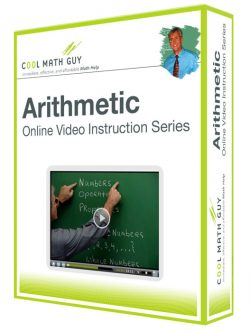# Arithmetic

### Course Info

Chapter 1 – WHOLE NUMBERS

• 1.1  Introduction to Whole Numbers
• 1.2  Addition of Whole Numbers
• 1.3  Subtraction of Whole Numbers
• 1.4  Multiplication of Whole Numbers
• 1.5  Division of Whole Numbers
• 1.6  Exponential Notation and the Order of Operations Agreement
• 1.7  Prime Numbers and Factoring

Chapter 2 – FRACTIONS

• 2.1  The least Common Multiple and Greatest Common Factor
• 2.2  Introduction to Fractions
• 2.3  Writing Equivalent Fractions
• 2.4  Addition of Fractions and Mixed Numbers
• 2.5  Subtraction of Fractions and Mixed Numbers
• 2.6  Multiplication of Fractions and Mixed Numbers
• 2.7  Division of Fractions and Mixed Numbers
• 2.8  Order, Exponents, and the Order of Operations Agreement

Chapter 3 – DECIMALS

• 3.1  Introduction to Decimals
• 3.2  Addition of Decimals
• 3.3  Subtraction of Decimals
• 3.4  Multiplication of Decimals
• 3.5  Division of Decimals
• 3.6  Comparing and Converting Fractions and Decimals

Chapter 4 – RATIO AND PROPORTION

• 4.1  Ratio
• 4.2  Rates
• 4.3  Proportions

Chapter 5 – PERCENTS

• 5.1  Introduction to Percents
• 5.2  Percent Equations: Part I
• 5.3  Percent Equations: Part II
• 5.4  Percent Equations: Part III
• 5.5  Percent Problems: Proportion Method

Chapter 6 – APPLICATIONS FOR BUSINESS AND CONSUMERS

• 6.1  Applications to Purchasing
• 6.2  Percent Increase and Percent Decrease
• 6.3  Interest
• 6.4  Real Estate Expenses
• 6.5  Car Expenses
• 6.6  Wages
• 6.7  Bank Statements

Chapter 7 – STATISTICS AND PROBABILITY

• 7.1  Pictographs and Circle Graphs
• 7.2  Bar Graphs and Broken-Line Graphs
• 7.3  Histograms and Frequency Polygons
• 7.4  Statistical Measures
• 7.5  Introduction to Probability

Chapter 8 – U.S. CUSTOMARY UNITS OF MEASUREMENT

• 8.1  Length
• 8.2  Weight
• 8.3  Capacity
• 8.4  Time
• 8.5  Energy and Power

Chapter 9 – THE METRIC SYSTEM OF MEASUREMENT

• 9.1  Length
• 9.2  Mass
• 9.3  Capacity
• 9.4  Energy
• 9.5  Conversion Between the U.S. Customary and the Metric Systems of Measurement

Chapter 10 – RATIONAL NUMBERS

• 10.1  Introduction to Integers
• 10.2  Addition and Subtraction of Integers
• 10.3  Multiplication and Division of Integers
• 10.4  Operations with Rational Numbers
• 10.5  Scientific Notation and the Order of Operations Agreement

Chapter 11 – INTRODUCTION TO ALGEBRA

• 11.1  Variable Expressions
• 11.2  Introduction to Equations
• 11.3  General Equations: Part I
• 11.4  General Equations: Part II
• 11.5  Translating Verbal Expressions into Mathematical Expressions
• 11.6  Translating Sentences into Equations and Solving

Chapter 12 – GEOMETRY

• 12.1  Angles, Lines, and Geometric Figures
• 12.2  Plane Geometric Figures
• 12.3  Area
• 12.4  Volume
• 12.5  The Pythagorean Theorem
• 12.6  Similar and Congruent Triangles

Course Access Options

•Select options (function(\$) { \$( '.products .post-2512 a' ).click( function() { if ( true === \$(this).hasClass( 'add_to_cart_button' ) ) { return; } ga( 'ec:addProduct', { 'id': '2512', 'name': 'Arithmetic - Full Course', 'category': 'Arithmetic/Full Courses', 'position': '1' }); ga( 'ec:setAction', 'click', { list: 'Product List' }); ga( 'send', 'event', 'UX', 'click', ' Product List' ); }); })(jQuery);

### Arithmetic – Full Course

From: \$19.95 / month with 1 day free trial
•Add to shopping bag (function(\$) { \$( '.products .post-2663 a' ).click( function() { if ( true === \$(this).hasClass( 'add_to_cart_button' ) ) { return; } ga( 'ec:addProduct', { 'id': '2663', 'name': 'Arithmetic - Educators Rate', 'category': 'Arithmetic/Educators Courses', 'position': '2' }); ga( 'ec:setAction', 'click', { list: 'Product List' }); ga( 'send', 'event', 'UX', 'click', ' Product List' ); }); })(jQuery);

\$50.00